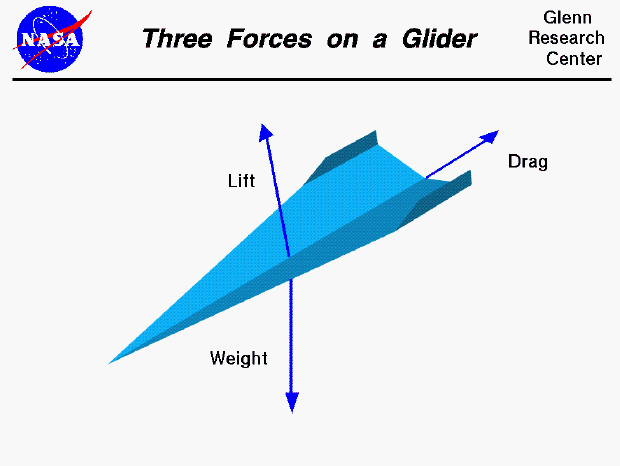# Effects of altitude on paper airplanes

If one were to fly a paper airplane at the Dead Sea (400 meters below sea level) and another identical paper airplane at the peak of Mount Everest (8800 meters above sea level) would there be any noticeable effects on the flight of the airplane? Assume still air with no thermals and flight far from surfaces such as the ground of rock formations.The effects I'm interested in are the differences in air pressure and temperature on the flight of a conventional paper airplane. Would humidity affect this as well? I've heard of how Reynolds number affects paper airplanes differently than jet airliners, and this number seems to depend on properties of the air as well as properties of the airplane.

Note that this question was translated into English by my father, who is the one typing it. I'm thirteen years old and I don't use SE unsupervised.

• Temperature and humidity should have negligible effect. Air density will have a huge effect, because lift is proportional to density and to velocity squared. So, for example, if density is reduced by a factor of 4, velocity has to increase by a factor of 2. Aug 22, 2020 at 1:27
• From this table it seems that the air density at the Dead Sea is about 24 someUnits and at the top of Everest about 18 someUnits. So that's about a 4/3 ratio of densities, so maybe about 1.15 ratio of velocities. But since the velocity is constant, by my hand, the plane will actually just have about 0.87 the amount of lift? Aug 23, 2020 at 9:01
• According to that table, density at 0 is 23.77, and at 30k is 8.91, so the density at 30k is .375 the density at sea level, so the velocity should be 1.63 times higher at 30k. And the speed of your hand is irrelevant. Assuming the airplane is nose-heavy (as normal airplanes have to be) it noses down until it reaches that speed. Aug 23, 2020 at 15:16

To answer this question, consider Bernouli's equation:

$$P= \frac{1}{2}\rho v^2$$

which is the pressure the wind will exert on the wings to generate lift. So how would the paper plane perform? The faster you throw the plane (the faster the wind speed below and above the plane, and assuming the existing wind speeds are negligible) will determine how fast and how long the plane will fly. If we rearrange this equation,

$$v = \sqrt \frac{2P}{\rho}$$

we can see that (how fast you throw the plane $$v$$ increasing the performance is obvious) the value of the pressure $$P$$ and density $$\rho$$ will be the determining factors. So what this means is if you were to throw the plane with the same strength at the Dead Sea (high pressure) as you would at the peak of Mount Everest (low pressure), we can see that the plane will perform much better at the Dead See. Of course a change in humidity would have an affect, but a change in humidity accompanies a change in pressure, so once again we are back to pressure being the important factor.

• Ohhh... You almost had it! What you need to do is equate $P$ with Lift (with a factor of area thrown in), and Lift is just the constant weight of the aircraft. So to get the same lift with lower density you need higher $v$, which is a good thing. That is why aircraft fly as high as possible, not as low as possible. Aug 22, 2020 at 10:49
• Yes. Aircraft also have propulsion. In this case we are considering a situation where there is none (after the plane leaves the hand). Remember, the person asking the question is 13 years old so we should try to keep this answer appropriate to the "average level of understanding" for someone that age. Aug 22, 2020 at 23:41
• Thank you. I was familiar with the concept but not the fomula for Bernoulli's principle. Aug 23, 2020 at 9:05
• @Drjh: Propulsion does not affect speed. It affects ascent or descent. A glider simply descends at the rate that gives it the natural speed. In fact, that's the only way airplanes can get down to land. The natural speed is determined by the amount of up-trim. Aug 23, 2020 at 15:20
• @Drjh: Here's how you establish up-trim in a paper airplane. At the trailing edge of the wing, bend the wing up, or cut a little tab and bend that up. That will make the airplane go slower (and stay up longer). Bend it straight or down, and the airplane will go faster (and descend faster). This is all assuming it is a bit nose-heavy. If it isn't nose-heavy it will follow a scalloped path - curving up, stalling, falling, and curving up again. Aug 25, 2020 at 11:28